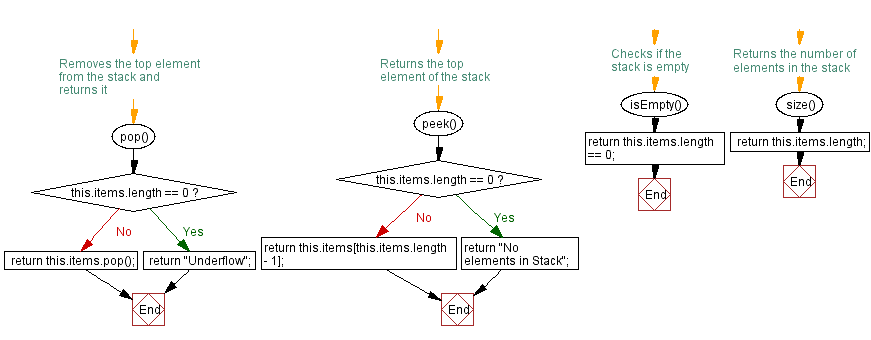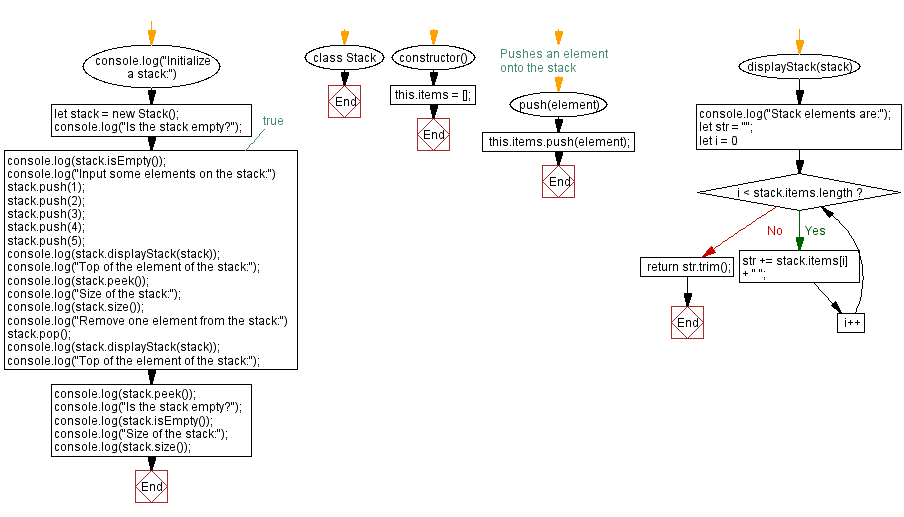# JavaScript Exercises: Implement a stack with push and pop operations

## JavaScript Stack: Exercise-1 with Solution

Write a JavaScript program to implement a stack with push and pop operations. Find the top element of the stack and check if it is empty or not.

Sample Solution:

JavaScript Code:

``````class Stack {
constructor() {
this.items = [];
}

// Pushes an element onto the stack
push(element) {
this.items.push(element);
}

// Removes the top element from the stack and returns it
pop() {
if (this.items.length == 0)
return "Underflow";
return this.items.pop();
}

// Returns the top element of the stack
peek() {
if (this.items.length == 0)
return "No elements in Stack";
return this.items[this.items.length - 1];
}

// Checks if the stack is empty
isEmpty() {
return this.items.length == 0;
}

// Returns the number of elements in the stack
size() {
return this.items.length;
}

displayStack(stack) {
console.log("Stack elements are:");
let str = "";
for (let i = 0; i < stack.items.length; i++)
str += stack.items[i] + " ";
return str.trim();
}
}
console.log("Initialize a stack:")
let stack = new Stack();
console.log("Is the stack empty?");
console.log(stack.isEmpty()); // true
console.log("Input some elements on the stack:")
stack.push(1);
stack.push(2);
stack.push(3);
stack.push(4);
stack.push(5);
console.log(stack.displayStack(stack));
console.log("Top of the element of the stack:");
console.log(stack.peek());
console.log("Size of the stack:");
console.log(stack.size());
console.log("Remove one element from the stack:")
stack.pop();
console.log(stack.displayStack(stack));
console.log("Top of the element of the stack:");
console.log(stack.peek());
console.log("Is the stack empty?");
console.log(stack.isEmpty());
console.log("Size of the stack:");
console.log(stack.size());
```
```

Sample Output:

```Initialize a stack:
Is the stack empty?
true
Input some elements on the stack:
Stack elements are:
1 2 3 4 5
Top of the element of the stack:
5
Size of the stack:
5
Remove one element from the stack:
Stack elements are:
1 2 3 4
Top of the element of the stack:
4
Is the stack empty?
false
Size of the stack:
4
```

Flowchart:Live Demo:

See the Pen javascript-stack-exercise-1 by w3resource (@w3resource) on CodePen.

Improve this sample solution and post your code through Disqus

Stack Previous: JavaScript Stack Exercises Home.
Stack Exercises Next: Sort the elements of a stack in descending order.

What is the difficulty level of this exercise?

Test your Programming skills with w3resource's quiz.

﻿